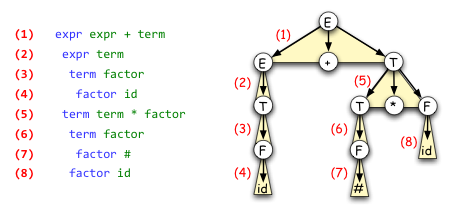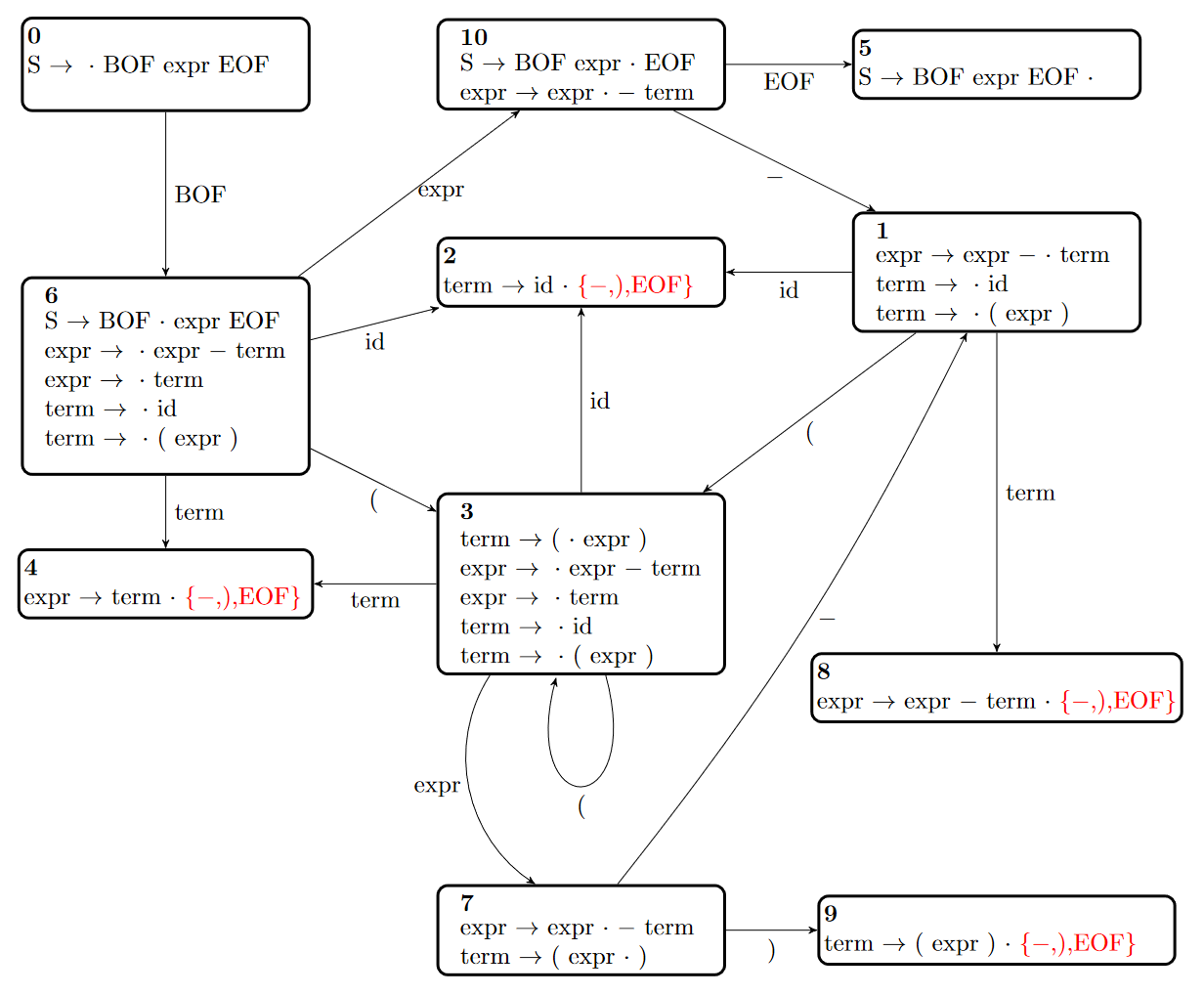# Context-Free Grammar & Parsing File Formats

The assignment on context-free grammars and parsing involves various file formats, which are constructed by combining various components. These components are summarized below.

## CFG Component

A CFG component starts with a header line `.CFG`, followed by a sequence of lines representing the rules of the CFG. Each line after the header has the form:

```left-hand-side-symbol right-hand-side-symbols
```

Where `left-hand-side-symbol` is a single symbol, and `right-hand-side-symbols` is either a sequence of symbols separated by whitespace, or the special string `.EMPTY`. A symbol is a string of printable non-whitespace ASCII characters that is not equal to `.EMPTY`.

These lines should be interpreted as CFG production rules. For example, the line `expr expr - term` would represent the rule `expr → expr - term`.

The special string `.EMPTY` represents the empty string (normally denoted ε); so the line `S .EMPTY` would represent the rule `S → ε`.

The other elements of the CFG may be inferred from the production rules as follows:

• The `left-hand-side-symbol` of the first line after the header is the start symbol.
• A symbol is a nonterminal symbol if and only if it appears as the `left-hand-side-symbol` of some rule.
• Therefore, the symbols which only appear on the right hand sides of rules are the terminal symbols.

### Example

Consider the following CFG with start symbol `expr`:

```expr → expr + term | term
term → term * factor | factor
factor → # | id
```

As a CFG component, it would look like this:

```.CFG
expr expr + term
expr term
term term * factor
term factor
factor #
factor id
```

Here is an example that uses the empty string:

```S → (S)S | ε
```

As a CFG component, it would look like this:

```.CFG
S ( S ) S
S .EMPTY
```

Note the whitespace in the first rule. If we wrote it as `S (S)S`, then `(S)S` would be treated as a single symbol instead of four symbols.

## DERIVATION Component

A DERIVATION component starts with a header line `.DERIVATION`, and is followed by a sequence of lines representing the steps of a leftmost derivation of a string, using the rules of the specified CFG.

Each line contains a single rule from the CFG, representing the rule that was applied to expand the leftmost nonterminal at that step the derivation. These rule lines have the same format as the rule lines in a CFG component.

The derivation steps must satisfy the following conditions to be valid:

• The first step must be a rule with the start symbol of the CFG on the left hand side.
Define the first sentence to be the right hand side of this rule.
• For n > 1, the n-th step must be a rule such that the left hand side of the rule matches the leftmost nonterminal symbol in the (n-1)-th sentence.
Define the n-th sentence to be the sequence obtained by replacing this leftmost nonterminal symbol with the right hand side of the rule.
• If there are m total derivation steps, then the m-th sentence should be a (possibly empty) sequence consisting only of terminal symbols.

The derivation steps can optionally be preceded by whitespace. This allows you to use indentation to organize your derivations, which you may find useful.

### Example

Suppose this is our CFG component:

```.CFG
expr expr + term
expr term
term term * factor
term factor
factor #
factor id
```

Here is a DERIVATION component for this CFG:

```.DERIVATION
expr expr + term
expr term
term factor
factor id
term term * factor
term factor
factor #
factor id
```

The indentation is optional, but makes it a little easier to see which line expands which nonterminal. This represents the following leftmost derivation:

```expr
⇒ expr + term
⇒ term + term
⇒ factor + term
⇒ id + term
⇒ id + term * factor
⇒ id + factor * factor
⇒ id + # * factor
⇒ id + # * id```

The image below shows the correspondence between the leftmost derivation and the parse tree for the string `id + # * id`.## INPUT Component

An INPUT component starts with a header line `.INPUT`, followed by a sequence of terminal symbols from the CFG, with each symbol separated by whitespace (possibly including newlines). This represents an input string for a parser. The parser should interpret the entire input section as a single string to be parsed, not as multiple strings.

### Example

Suppose we have this CFG component:

```.CFG
S BOF expr EOF
expr expr - term
expr term
term id
term ( expr )
```

Each of the following INPUT components is a valid way of specifying the following input sequence: `BOF id - ( id - id ) EOF`

```.INPUT
BOF id - ( id - id ) EOF

.INPUT
BOF
id - ( id - id )
EOF

.INPUT
BOF
id
-
(
id
-
id
)
EOF
```

## ACTIONS Component

An ACTIONS component starts with a header line `.ACTIONS`, and is followed by a sequence of lines representing actions for an bottom-up parser to perform. Each line has one of the following forms:

```shift
reduce n
print
```

In a `reduce n` line, `n` is a non-negative integer referring to a rule in the CFG. The first rule is numbered 0, the second rule is numbered 1, and so on.

See the assignment specification for more details on how the actions in an ACTIONS component should be processed.

## TRANSITIONS Component

A TRANSITIONS component starts with a header line `.TRANSITIONS`, followed by a sequence of lines encoding the transitions of an SLR(1) parsing DFA for the given CFG.

States of the DFA are represented by non-negative integers, and the initial state of the DFA is state 0.

To completely specify an SLR(1) parsing DFA, a REDUCTIONS component (discussed below) is also required.

Each line after the header has the form:

```from-state symbol to-state
```

Where `from-state` and `to-state` are non-negative integers, and `symbol` is a terminal or nonterminal symbol from the CFG.

## REDUCTIONS Component

A REDUCTIONS component starts with a header line `.REDUCTIONS`, followed by a sequence of lines encoding information about the reducible items in the SLR(1) parsing DFA.

To completely specify an SLR(1) parsing DFA, a TRANSITIONS component (discussed above) is also required.

Each line after the header has the form:

```state-number rule-number tag
```

Where `state-number` and `rule-number` are non-negative integers, and `tag` is either a terminal symbol from the CFG, or the special string `.ACCEPT`. Each of these lines has the following meaning:

• State `state-number` contains a reducible item corresponding to rule `rule-number`, where the rules are numbered in the order they appear in the CFG section, starting from 0.
• If `tag` is a terminal symbol, this symbol is one of the "lookahead tags" for the reducible items. That is, it is an elements of the Follow set of the left-hand-side of the item's rule.
• If `tag` is the special string `.ACCEPT`, this indicates that the parser can only reach this state after all input is consumed, and the input string should be accepted after this reduction.
• You may assume that the special string `.ACCEPT` is never a terminal in the CFG.

### Example

Consider the following CFG:
```S → BOF expr EOF
expr → expr - term
expr → term
term → id
term → ( expr )
```

The components below encode this CFG and its SLR(1) DFA.

 ```.CFG S BOF expr EOF expr expr - term expr term term id term ( expr ) .TRANSITIONS 0 BOF 6 1 id 2 1 ( 3 1 term 8 3 id 2 3 ( 3 3 term 4 3 expr 7 6 id 2 6 ( 3 6 term 4 6 expr 10 7 - 1 7 ) 9 10 - 1 10 EOF 5 .REDUCTIONS 2 3 - 2 3 ) 2 3 EOF 4 2 - 4 2 ) 4 2 EOF 5 0 .ACCEPT 8 1 - 8 1 ) 8 1 EOF 9 4 - 9 4 ) 9 4 EOF ```## .END

This is not a true component; it is simply used to mark end of a file containing components. Every input file format used on the assignment will end with a line that just contains `.END` so that when you read this line, you know you are done reading the file.